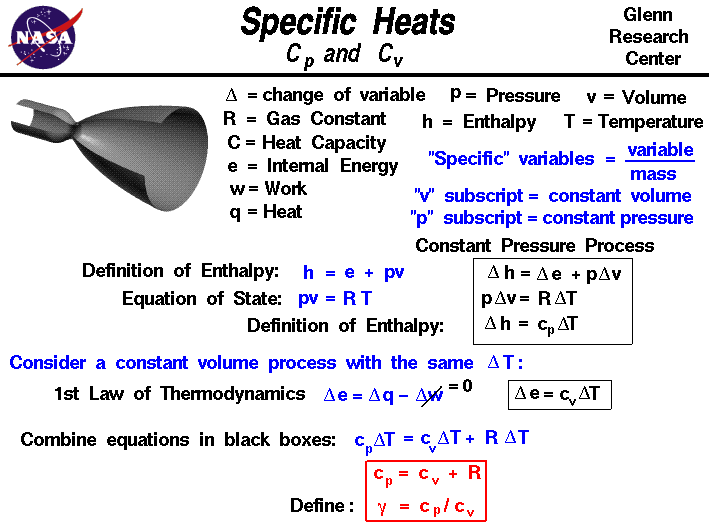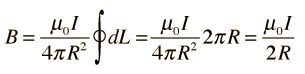Home » 2016 » February

# Monthly Archives: February 2016

## Physics and Mathematicshello sir
This is anurag choudhary. I am a post BSc student of Physics . I have special liking for science and mathematics in all  its aspects. In free time I think about general  relations of  science and in this pursuit I have written a short thought  question that I want to share with you.  My question   and explanation are as explained below.
Thinking is a common and continuous process for all of us. Now I have a question, “have you ever thought whether thinking is reversible or irreversible process. let us try to understand  it. suppose somebody asks us about components of knowledge so  far as fact based knowledge is concerned, one can discuss about science, mathematics,humanity and commerce. if we  ask a particular person belonging to above stream they will  answer  according to their stream or what they have learnt in their text. Even in public life  we discuss an  event related to past and then come to conclusion so actually  we are making conclusions based on no of references we have. what does reference mean here ? Reference here mean how many past experiences we have? see what we think today is a reflection of our next day approach so we can say our present day thinking is a function of our past thinking and future is extension of coming past i.e today’s present so our present past and future is related to our thinking
process and we can conclude our present and future is an extension of past and it is the past which relates present
and future. thus we can conclude our thinking process is reversible. also we can think as future and present are forms of past so we can say thinking process is a mathematical function and that function is symmetric about past as origin
and also we can say time is reversible in nature as our everyday discussion starts with past and again same past is repeated isn’t this mean past is a composite vector space and our life is discussed as its components i.e subspaces. and also we can say past is a vector space and present and future are its subspaces.Now what i mean to express from
past experiences is that past is a reference frame for time to make present day observation. Have we ever thought of any thinking without reference frame of time? or have we ever thought why past repeats as cyclic representation of vector spaces? it is basically our thinking process which repeats and it went through same angle and curvature at particle time so can we think is this the source of relativity of nature?

## Why gases have 2 specific heats?

Why gases have 2 specific heats?Akhil Francis Specific heat capacity at constant pressure and specific heat capacity at constant volume……It is because heat energy supplied at constant Pressure changes both Internal Energy and also does Some amount of work…..while Heat energy supplied at constant volume only changes it’s internal energy and does not do any work as Volume is a constant…

A simple relation between two specific heat capacities are given by

Cp – Cv=R

## what to study in atoms and nuclei chapter for AISSCE?

What to study in atoms and nuclei chapter for AISSCE?As per CBSE Syllabus the contents are

### Chapter–12: Atoms

Alpha-particle scattering experiment; Rutherford’s model of atom; Bohr model, energy levels, hydrogen spectrum.

### Chapter–13: Nuclei

Composition and size of nucleus, Radioactivity, alpha, beta and gamma particles/rays and their properties; radioactive decay law.
Mass-energy relation, mass defect; binding energy per nucleon and its variation with mass number; nuclear fission, nuclear fusion.

Though all portions are to be learnt, the topics given in bold above needs more attention.

Have a look at the following questions which are frequently asked from the unit. These questions are collected from previous question papers

1. Why is it found experimentally difficult to detect neutrinos in nuclear
beta -decay ?
2. Using Rutherford model of the atom, derive the expression for the total energy of the electron in hydrogen atom. What is the significance of total negative energy possessed by the electron ?
3. Using Bohr’s postulates of the atomic model, derive the expression for radius of nth electron orbit. Hence obtain the expression for Bohr’s radius.
4. The figure shows energy level diagram of hydrogen atom.
(a) Find out the transition which results in the emission of a photon of wavelength
496 nm.
n = 4
n = 3
n = 2
n = 1
(b) Which transition corresponds to the emission of radiation of maximum
5. (a) Complete the following nuclear reactions :
(i) 208Po84 –>  204Pb82 + …
(ii) 32P 15 –> 32S16 + …
(b) Write the basic process involved in nuclei responsible for (i) beta– and (ii) beta+ decay.
(c) Why is it found experimentally difficult to detect neutrinos ?
6. (a) Write the relation for binding energy (BE) (in MeV) of a nucleus of
mass M, atomic number (Z) and mass number (A) in terms of the
masses of its constituents — neutrons and protons.
(b) Draw a plot of BE/A versus mass number A for 2 <= A <= 170. Use
this graph to explain the release of energy in the process of nuclear
fusion of two light nuclei.ALSO PRACTICE

1. Describe Rutherford’s alpha scattering experiment with neat labelled diagram and write the conclusions drawn from the result of experiment.
2. Derive an expression for the distance of closest approach in Rutherford’s alpha scattering experiment.
3. State postulates of Bohr atom model.
4. What are the drawbacks of Rutherford’s model of atom?
5. Describe the formula for spectral series of hydrogen atom and draw diagram showing the transitions resulting in various spectral series of hydrogen.
6. State the properties of nuclear force.
7. Draw graph showing the variation of Binding Energy per nucleon and explain the release of energy during nuclear fission and fusion using the graph.
8. Show that the nuclear density is independent of mass number.
10. Define half-life of a radioactive element and derive an expression for it in terms of decay constant.

## matter, anti-matter

I was watching a physics show on television the other night.  It involved the first second after the big bang.  They discussed the process by which energy transmuted into matter and that both matter and anti-matter were created. They seemed to marvel at the idea that the number of particles of matter must have outnumbered the number of anti-matter particles by only one in a billion. And the result of the remaining particles of matter accounted for the entire universe that we see today. And since matter and anti-matter annihilate each other upon contact and become energy, my question is this….is the energy created by their annihilation energy or anti-energy? And if it was energy, then why would it not be possible for it to transmute into matter again?  Thus accounting for the predominance of matter versus anti-matter.  And what the hell is TIME?  Is it some weird form of energy or what? (only kidding about the “time” question)

Posted by  John Hayes

## Car in a negative super elevation roundabout

“Can some one draw the free body diagram for a car travelling in a negative superelevation roundabout with friction considered? ?  What is the max and min speed for not skidding??”## space flight propulsion

I understand that space in a vacuum, and is extremely cold. I understand that to push something trough space such as a rocket you need to create a force. Now I’ve researched many new technologies such as ion propulsion, nuclear antimatter reactors, etc. But they all still require a fuel. I want to know if it is possible to make two magnetic fields oppose each other in a chamber then harness the heat and use that a a propulsion. Also another though is can solar rays be harnessed and produce gamma radiation and use that as a force?

Posted Ethan Appleton

## calculating number of turns

How to determine the number of turns needed to generate specific magnetic field?
my condition is that the area of the magnet field strength must be able to sense a magnet material which is placed 4meters away from it. And voltage applied is 2.6v. Is there any formula?

The question is not very clear. Is it a single loop whose area is to be determined or whether the area is given and no. of turns to be calculated?

Anyway, I am giving here the formulae.

If, V is given, then current can be calculated if R is given.Use this online calculator to solve your question, if you have necessary data.

http://hyperphysics.phy-astr.gsu.edu/hbase/magnetic/curloo.html

If any clarifications required please post as comment to this post.